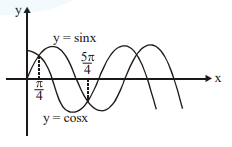# The graphs of sine and cosine functions,

Question:

The graphs of sine and cosine functions, intersect each other at a number of points and between two consecutive points of intersection, the two graphs enclose the same area $A$. Then $\mathrm{A}^{4}$ is equal to______.

Solution:$A=\int_{\pi / 4}^{5 \pi / 4}(\sin x-\cos x) d x$

$=\left.(-\cos x-\sin x)\right|_{\sqrt / 4} ^{5 \pi / 4}$

$=\left(-\left(\frac{-1}{\sqrt{2}}\right)-\left(\frac{-1}{\sqrt{2}}\right)\right)-\left(-\left(\frac{1}{\sqrt{2}}\right)-\left(\frac{1}{\sqrt{2}}\right)\right)$

$\Rightarrow A=\frac{2}{\sqrt{2}}+\frac{2}{\sqrt{2}}=2 \sqrt{2}$

$\Rightarrow \mathrm{A}^{4}=(2 \sqrt{2})^{4}=16 \times 4=64$